Courses

# Design of Helical Springs for Variable Load - 1 Notes | EduRev

## Mechanical Engineering : Design of Helical Springs for Variable Load - 1 Notes | EduRev

The document Design of Helical Springs for Variable Load - 1 Notes | EduRev is a part of the Mechanical Engineering Course Design of Machine Elements.
All you need of Mechanical Engineering at this link: Mechanical Engineering

Design of helical spring for variable load

In the earlier lecture, we have learned about design of helical springs for static loads. In many applications, as for example in railway carriages or in automobile suspension systems the helical springs used are constantly under variable load. Hence, it is understood that whenever there is a variable load on a spring the design procedure should include the effect of stress variation in the spring wire. The methodology used is the modified Soderberg method. we have learnt about Soderberg method in earlier chapter, here, the necessary modifications applicable to helical spring design will be discussed.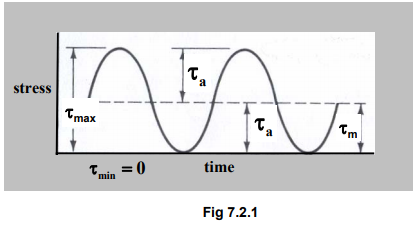From Fig.7.2.1 we see that ,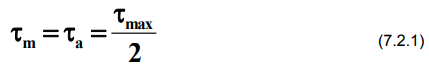Where, τa is known as the stress amplitude and τm is known as the mean stress or the average stress. We know that for varying stress, the material can withstand stress not exceeding endurance limit value. Hence, for repeated torsion experiment, the mean stress and the stress amplitude become,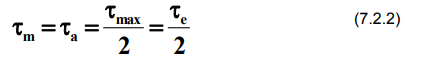Soderberg failure criterion

The modified Soderberg diagram for repeated stress is shown in the Fig 7.2.2.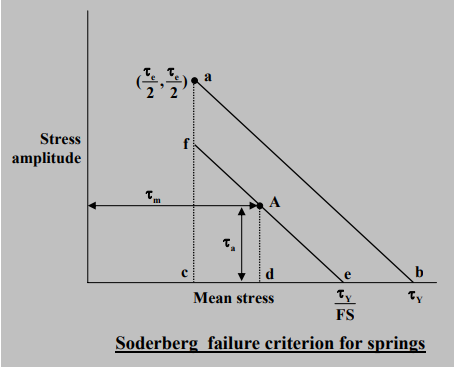Fig 7.2.2

The stress being repeated in nature, the co-ordinate of the point a is , 2 2 e e τ τ . For safe design, the design data for the mean and average stresses, τa and τm respectively, should be below the line a-b. If we choose a value of factor of safety (FS), the line ab shifts to a newer position as shown in the figure. This line e-f in the figure is called a safe stress line and the point A ( , τm, τa ) is a typical safe design point.

Considering two similar triangles, abc and Aed respectively, a relationship between the stresses may be developed and is given as,Considering two similar triangles, abc and Aed respectively, a relationship between the stresses may be developed and is given as,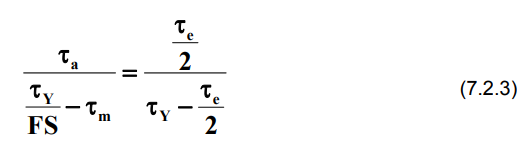where τY is the shear yield point of the spring material.

In simplified form, the equation for Soderberg failure criterion for springs is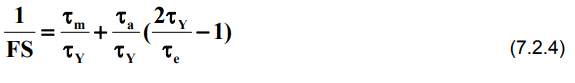The above equation is further modified by considering the shear correction factor, Kand Wahl correction factor, Kw. It is a normal practice to multiply τm by Ks and to multiply τa by Kw.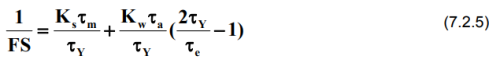The above equation for Soderberg failure criterion for will be utilized for the designing of springs subjected to variable load.

Estimation of material strength

It is a very important aspect in any design to obtain correct material property. The best way is to perform an experiment with the specimen of desired material. Tensile test experiments as we know is relatively simple and less time consuming. This experiment is used to obtain yield strength and ultimate strength of any given material. However, tests to determine endurance limit is extremely time consuming. Hence, the ways to obtain material properties is to consult design data book or to use available relationships, developed through experiments, between various material properties. For the design of springs, we will discuss briefly, the steps normally used to obtain the material properties. One of the relationships to find out ultimate strength of a spring wire of diameter d is,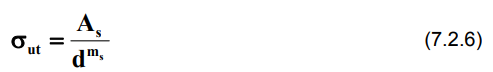For some selected materials, which are commonly used in spring design, the values of As and ms are given in the table below.

As                              ms

Hard-drawn wire               1510                     0.201
Oil-tempered wire             1610                     0.193
Chrome-silicon wire         1960                      0.091
Music wire                        2060                      0.163

The above formula gives the value of ultimate stress in MPa for wire diameter in mm. Once the value of ultimate strength is estimated, the shear yield strength and shear endurance limit can be obtained from the following table developed through experiments for repeated load.

Wire Type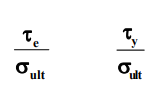Hard-drawn wire                0.21               0.42

Oil-tempered wire              0.22               0.45

Chrome-silicon wire           0.20               0.51

Music wire                          0.23             0.40
|302 SS wire                      0.20              0.46

Hence, as a rough guideline and on a conservative side, values for shear yield point and shear endurance limit for major types of spring wires can be obtained from ultimate strength as,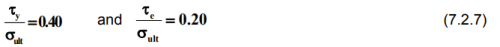With the knowledge of material properties and load requirements, one can easily utilize Soderberg equation to obtain spring design parameters.

Types of springs

There are mainly two types of helical springs, compression springs and extension springs. Here we will have a brief look at the types of springs and their nomenclature.

Compression springs

Following are the types of compression springs used in the design

(a) Plain ends

Total coils, NT            : N

Solid length, LS         : d ( NT + 1 )

Free length, L       : LS +  δ max  +  δallowan

Pitch, p              : ( L – d ) / N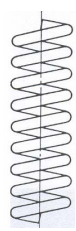Plain end spring Fig 7.2.3

In the above nomenclature for the spring, N is the number of active coils, i.e., only these coils take part in the spring action. However, few other coils may be present due to manufacturing consideration, thus total number of coils, NT may vary from total number of active coils.

Solid length, LS is that length of the spring, when pressed, all the spring coils will clash with each other and will appear as a solid cylindrical body. The spring length under no load condition is the free length of a spring. Naturally, the length that we visualise in the above diagram is the free length.

Maximum amount of compression the spring can have is denoted as δmax, which is calculated from the design requirement. The addition of solid length and the δmax should be sufficient to get the free length of a spring. However, designers consider an additional length given as δ allowance. This allowance is provided to avoid clash between to consecutive spring coils. As a guideline, the value of δ allowance is generally 15% of δmax.

The concept of pitch in a spring is the same as that in a screw.

(b) Plain and Ground ends

Total coils, NT              : N + 1
Solid length, LS          : d ( N)
Free length,         LS + +δ maxallowance
Pitch, p                              : L / ( N + 1)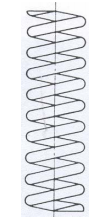Plain and Ground end spring Fig 7.2.4

The top and bottom of the spring is grounded as seen in the figure. Here, due to grounding, one total coil is inactive.

(c) Squared or closed ends

Total coils, NT              : N + 2
Solid length, LS          : d ( NT + 1 )
Free length, L             : LS + δmax + δallowance
Pitch, p                        : ( L - 3d ) / N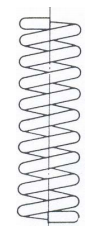Squared or closed end spring Fi g 7.2.5

In the Fig 7.2.5 it is observed that both the top as well as the bottom spring is being pressed to make it parallel to the ground instead of having a helix angle. Here, it is seen that two full coils are inactive.

(d) Squared and ground ends
Total coils, N                : N + 2
Solid length, LS              : d ( NT )
Free length, L                  LS + δmax + δallowance
Pitch, p                              : ( L - 2d ) / N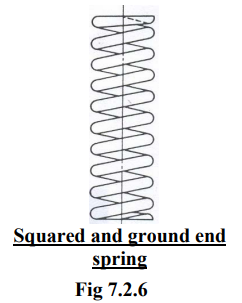It is observed that both the top as well as the bottom spring, as earlier one, is being pressed to make it parallel to the ground, further the faces are grounded to allow for proper seat. Here also two full coils are inactive.

Offer running on EduRev: Apply code STAYHOME200 to get INR 200 off on our premium plan EduRev Infinity!

## Design of Machine Elements

51 videos|62 docs|83 tests

,

,

,

,

,

,

,

,

,

,

,

,

,

,

,

,

,

,

,

,

,

;